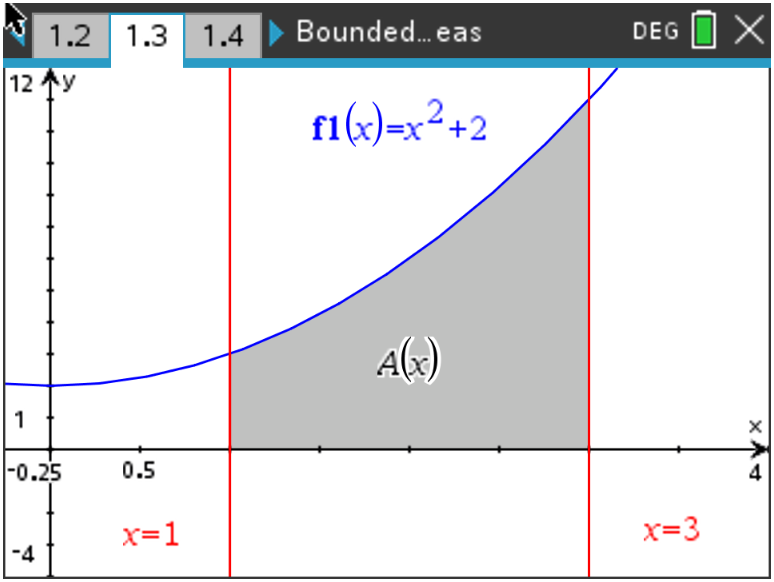### Mathematics lessons for IB® Diploma Programme

Applications and Interpretation | Calculus

# Bounded Areas### Activity Overview

In this lesson, students will compare the Riemann Sum area estimates with the exact bounded area of a curve and the x-axis.

### Objectives

• Students will practice and discuss applications of finding the area bounded by a curve and the x-axis.
• Students will apply this information to finding the area bounded by two curves.
• Students will try to make a connection with how to understand these topics in both IB Mathematics and AP Calculus and on their final assessments.

### Vocabulary

 Riemann Sums Underestimate Overestimate Definite Integrals Bounded Areas Concavity